# Swift-枚举&Optional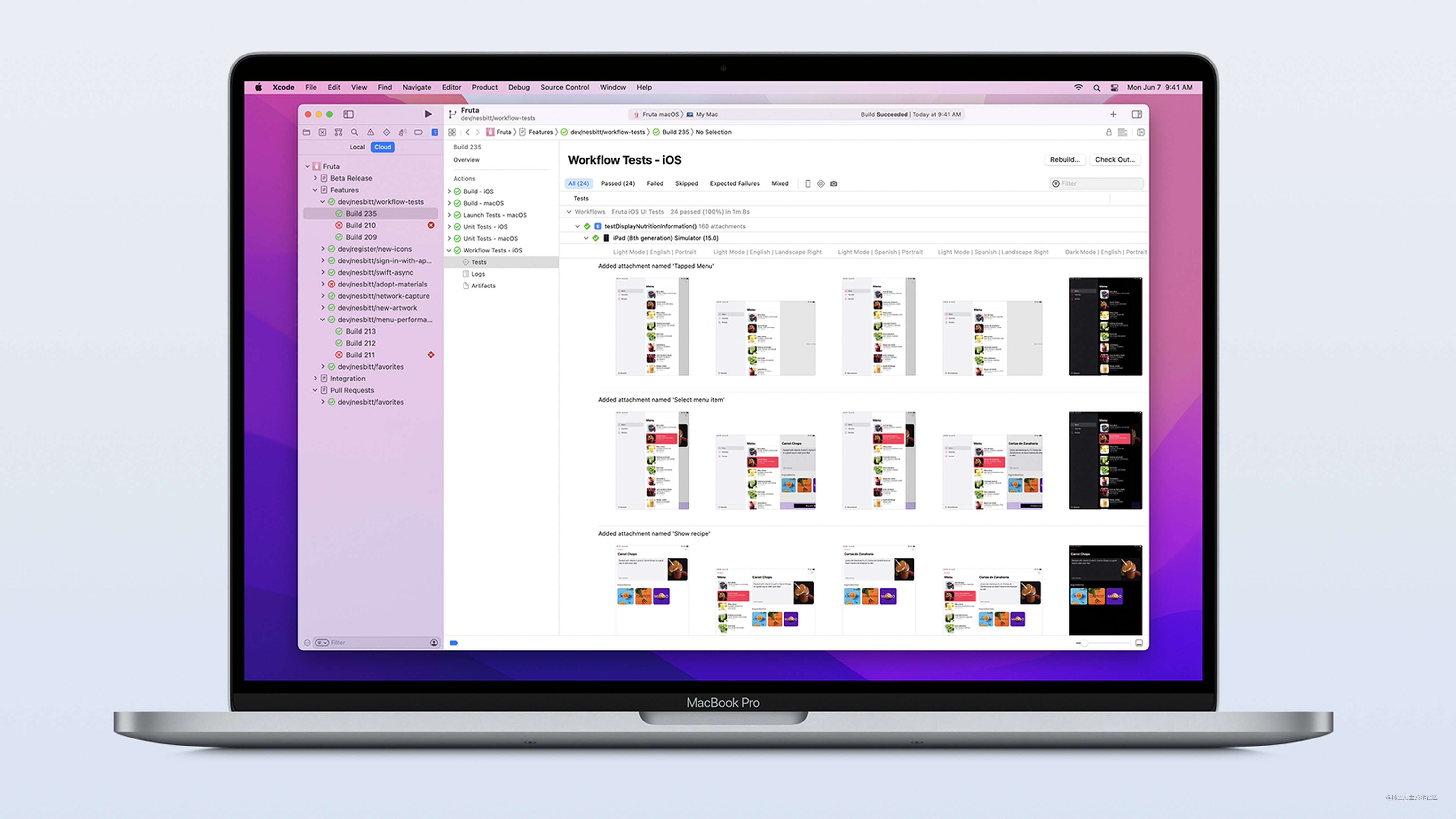`枚举`为一组相关的值定义了一个共同的类型，使你可以在代码中以类型安全的方式来使用这些值。在`C`或者`Objective-C`语言中，枚举会为一组整型值分配相关联的名称。

### 枚举

#### 枚举的基本用法

`Swift`中的枚举则更加灵活，并且不需给枚举中的每一个成员都提供值。如果一个值(`原始值`)要被提供给每一个枚举成员，那么这个值可以是`字符串``字符`、任意的`整数值`，或者是`浮点类型`

``````enum ATEnum {
case one
case two
case three
}

``````typedef NS_ENUM(NSInteger, ATEnum) {
A,
B,
C
}

``````// String类型
enum Color: String {
case red = "Red"
case amber = "Amber"
case green = "Green"
}

// Double类型
enum LGEnum: Double {
case a = 10.0
case b = 20.0
case c = 30.0
case d = 40.0
}

``````enum DayOfWeek: Int {
case mon, tue, wed, thu, fri = 10, sat, sun
}

##### SIL文件分析

``````enum DayOfWeek: String {
case mon, tue, wed, thu, fri = "hello", sat, sun
}

var x = DayOfWeek.mon.rawValue

``````// 生成sil命令
swiftc -emit-sil main.swift > ./main.sil

``````enum DayOfWeek : String {
case mon, tue, wed, thu, fri, sat, sun
init?(rawValue: String) // 可失败初始化器
typealias RawValue = String // 取别名
var rawValue: String { get } // get rawValue函数
}

``````// main
sil @main : \$@convention(c) (Int32, UnsafeMutablePointer<Optional<UnsafeMutablePointer<Int8>>>) -> Int32 {
bb0(%0 : \$Int32, %1 : \$UnsafeMutablePointer<Optional<UnsafeMutablePointer<Int8>>>):
alloc_global @\$s4main1xSSvp                     // id: %2
%3 = global_addr @\$s4main1xSSvp : \$*String      // user: %8
%4 = metatype \$@thin DayOfWeek.Type
%5 = enum \$DayOfWeek, #DayOfWeek.mon!enumelt    // user: %7

// function_ref DayOfWeek.rawValue.getter (rawValue的getter方法)
%6 = function_ref @\$s4main9DayOfWeekO8rawValueSSvg : \$@convention(method) (DayOfWeek) -> @owned String // user: %7
%7 = apply %6(%5) : \$@convention(method) (DayOfWeek) -> @owned String // user: %8
store %7 to %3 : \$*String                       // id: %8
%9 = integer_literal \$Builtin.Int32, 0          // user: %10
%10 = struct \$Int32 (%9 : \$Builtin.Int32)       // user: %11
return %10 : \$Int32                             // id: %11
} // end sil function 'main'

`rawValue``getter`方法里，通过获取`mon`的枚举值传入到`getter`方法(也就是上面的`s4main9DayOfWeekO8rawValueSSvg`)，搜索`s4main9DayOfWeekO8rawValueSSvg`函数看一下它的定义：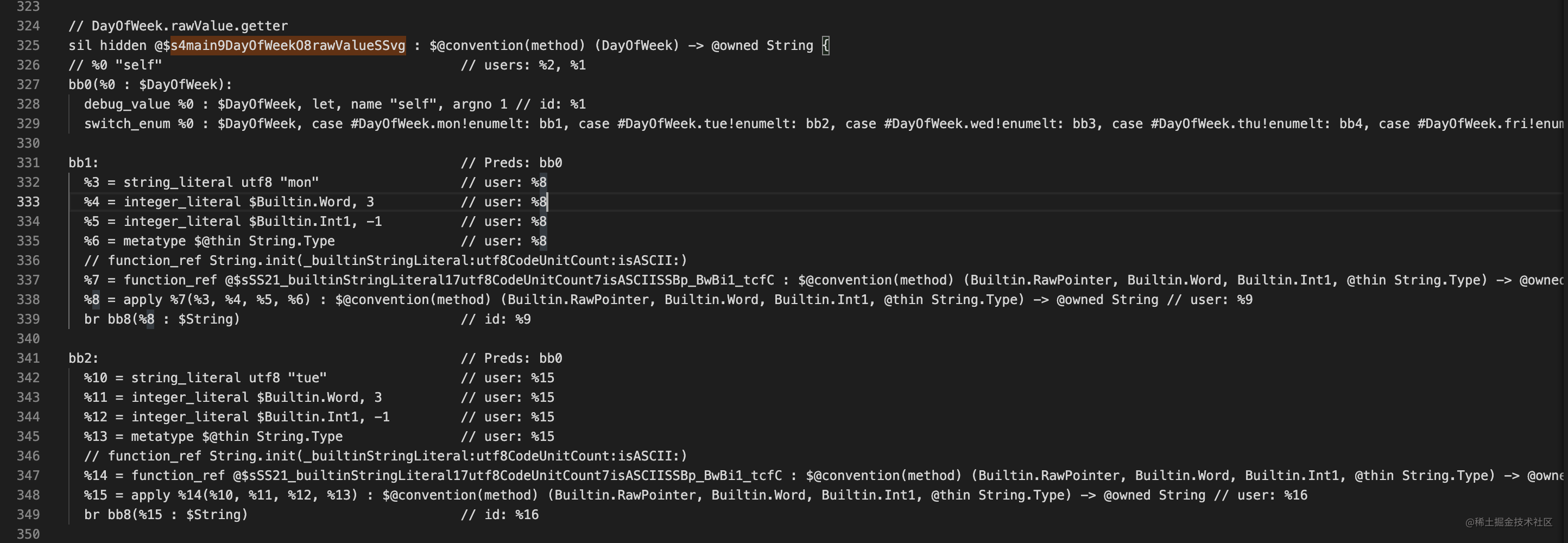• `self`就是`DayOfWeek`
• `%0`就是传进来的`枚举成员值`
• 通过模式匹配走到对应的分支(`bb1、bb2...`)
• 不同的代码分支里获取到对应的字符串给到不同分支的返回值 而上面的字符串其实就是从`Mach-O`文件的`Section64(__TEXT,__cstring)`里读取的。

#### 枚举值&原始值

``````DayOfWeek.init(rawValue: "mon")

#### 关联值

``````// 通过给定关联值来表示具体的图形
enum Shape {
case rectangle(width: Double, height: Double)
}

##### 关联值的使用

``````// 定义一个半径为10的圆形
var circle = Shape.circle(radius: 10.0)
// 定义一个宽高为10、20的矩形
var rectangle = Shape.rectangle(width: 10.0, height: 20.0)

##### 关联值注意事项

``````enum Weak: String {
case MONDAY
case TUEDAY
case WEDDAY
case THUDAY
case FRIDAY
case SATDAY
case SUNDAY
}

let currentWeak: Weak = Weak.MONDAY

// 通过switch匹配具体的分支
switch currentWeak {
case .MONDAY:
print(Weak.MONDAY.rawValue)
case .TUEDAY:
print(Weak.TUEDAY.rawValue)
case .WEDDAY:
print(Weak.WEDDAY.rawValue)
case .THUDAY:
print(Weak.THUDAY.rawValue)
case .FRIDAY:
print(Weak.FRIDAY.rawValue)
case .SATDAY:
print(Weak.SATDAY.rawValue)
case .SUNDAY:
print(Weak.SUNDAY.rawValue)
}

``````switch currentWeak{
case .SATDAY, .SUNDAY: print("Happy Day")
default : print("Sad Day")
}

``````enum Shape {
case rectangle(width: Double, height: Double)
}

let shape = Shape.circle(radius: 10.0)

switch shape {

case let .rectangle(width, height):
print("rectangle width:\(width),height\(height)")
}

`switch`语句中可以从上面通过关联值拿到`radius`的值然后打印出来。`case let`就是模式匹配的写法，除了这种写法还可以把`let`放在参数里面，相当于声明了一个临时变量去接收`radius`的值，代码如下：

``````switch shape {

case .rectangle(let width, let height):
print("rectangle width:\(width), height\(height)")
}

#### 枚举的大小

`Single-payload enums`的内存布局，字面的意思就是有一个负载的`enum`，比如下面的例子：

``````enum ATEnum {
case test_one(Bool)
case test_two
case test_three
case test_four
}

``````enum ATEnum {
case test_one(Int)
case test_two
case test_three
case test_four
}

``````// 这里定义了2个Bool类型
enum ATEnum {
case test_one(Bool)
case test_two(Bool)
case test_three
case test_four
}

#### 递归枚举

`递归枚举`是一种枚举类型，它有一个或多个枚举成员使用该枚举类型的实例作为关联值。使用递归枚举时，编译器会插入一个间接层。你可以在定义枚举前加上`indirect`关键字来表示该成员可递归。

``````// 示例代码，二叉树
indirect enum BinaryTree<T> {
case empty
case node(left: BinaryTree, value: T, right: BinaryTree)
}

``````enum BinaryTree<T> {
case empty
indirect case node(left: BinaryTree, value: T, right: BinaryTree)
}

### Optional

#### 认识可选值

``````class ATTeacher {
var age: Int?
}

``````var age: Int? = var age: Optional<Int>

``````@frozen
public enum Optional<Wrapped>: ExpressibleByNilLiteral {
case none
case some(Wrapped)
}

``````enum MyOptional<Value> {
case some(Value)
case none
}

``````func getOddValue(_ value: Int) -> MyOptional<Int> {
if value % 2 == 0 {
return .some(value)
} else {
return .none
}
}

``````for element in array {
let value = getOddValue(element)
switch value {
case .some(let value):
array.remove(at: array.firstIndex(of: value)!)
case .none:
print("vlaue not exist")
}
}

``````func getOddValue(_ value: Int) -> Int? {
if value % 2 == 0 {
return .some(value)
} else {
return .none
}
}

``````if let value = value {
array.remove(at: array.firstIndex(of: value)!)
}

1. `if let`方式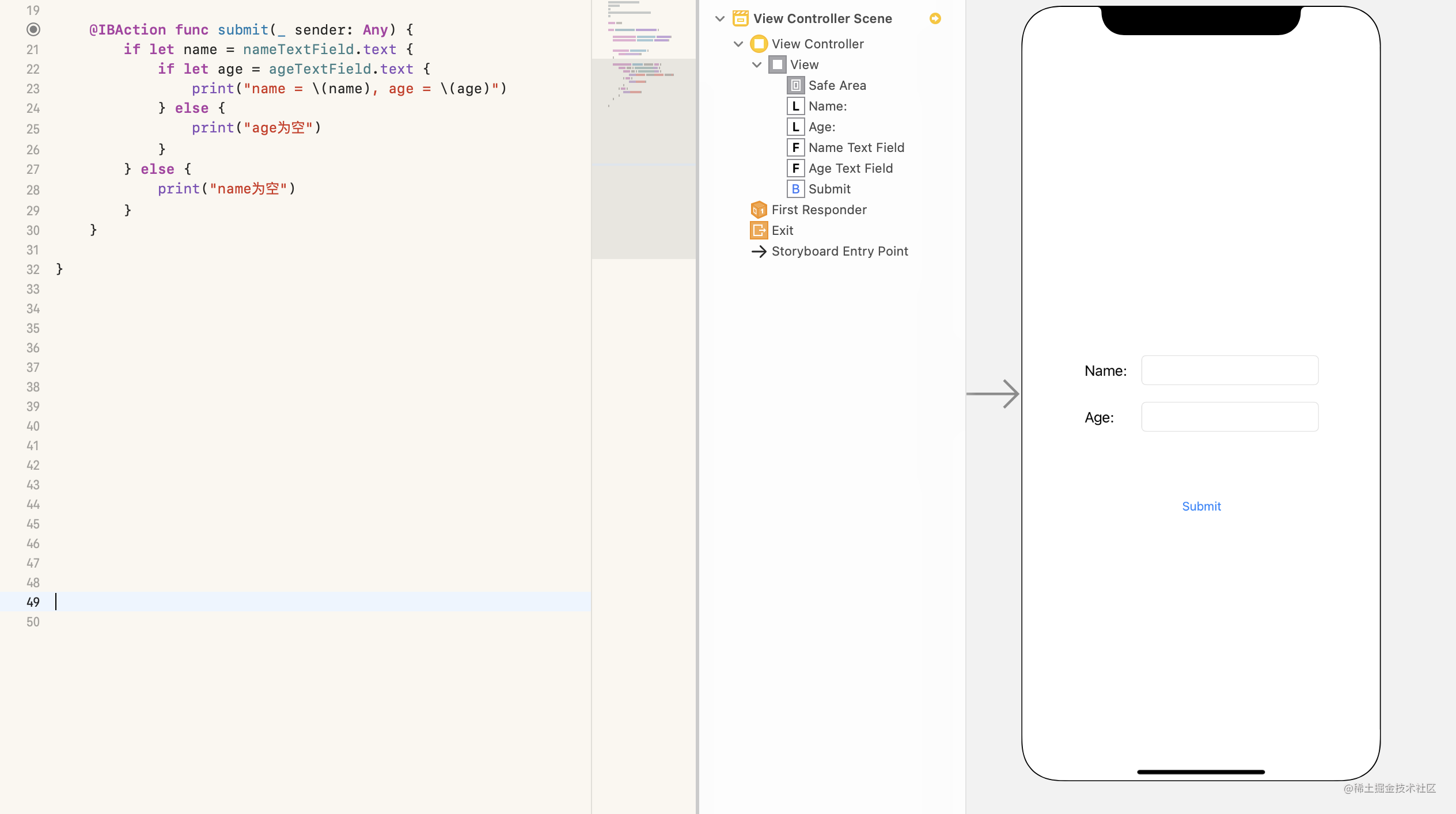2. `guard let`方式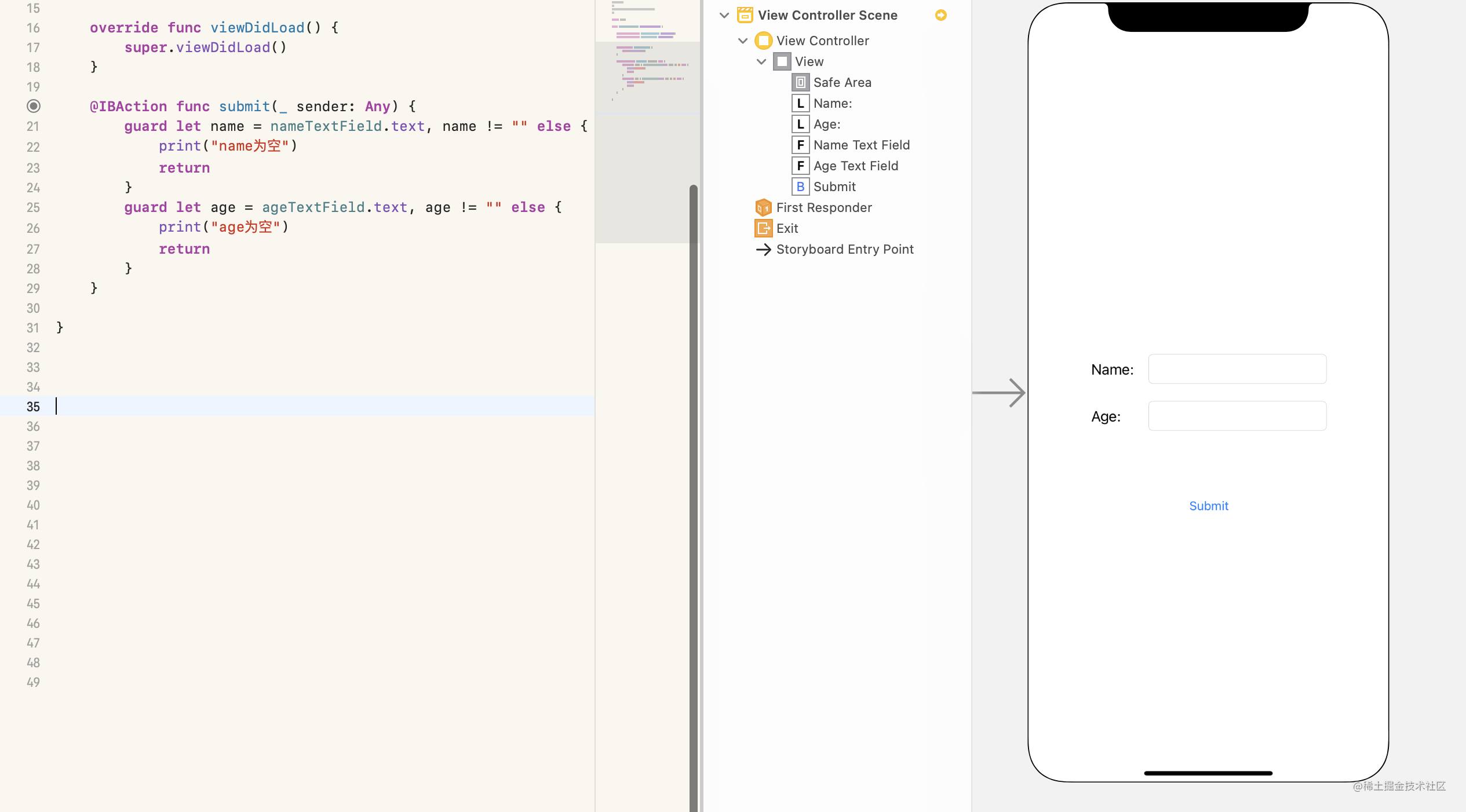• `guard let``if let`刚好相反，`guard let`守护一定有值，如果没有直接返回。
• 通常判断是否有值之后，会做具体的逻辑实现，通常代码多，如果用`if let`凭空多了一层分支，`guard let`是降低分支层次的办法。

#### 可选链

``````let str: String? = "abc"
let upperStr = str?.uppercased() // Optional<"ABC">
var str: String?
let upperStr = str?.uppercased() // nil

``````var closure: ((Int) -> ())?
closure?(1) // closure为nil不执行
let dict = ["one": 1, "two": 2]
dict?["one"] // Optional(1)
dict?["three"] // nil

#### ??运算符(空合并运算符)

`(a ?? b)`将对可选类型`a`进行空判断，如果`a`包含一个值就进行解包，否则就返回一个默认值`b`

• 表达式`a`必须是`Optional`类型
• 默认值`b`的类型必须要和`a`存储值的类型保持一致

#### 运算符重载

``````struct Vector {
let x: Int
let y: Int
}

extension Vector {
static func + (firstVector: Vector, secondVector: Vector) -> Vector {
return Vector(x: firstVector.x + secondVector.x, y: firstVector.y + secondVector.y)
}

static prefix func - (vector: Vector) -> Vector {
return Vector(x: -vector.x, y: -vector.y)
}

static func - (firstVector: Vector, secondVector: Vector) -> Vector {
return firstVector + -secondVector
}
}

##### 自定义运算符

``````infix operator operator

``````// 优先级组声明有以下形式
precedencegroup precedence group name {
higherThan: lower group names
lowerThan: higher group names
associativity: associativity
assignment: assignment
}

``````// 自定义乘法
infix operator &*: AdditionPrecedence

precedencegroup AtomPrecedence {
associativity: left // 左结合
}

``````extension Vector {
static func &* (firstVector: Vector, secondVector: Vector) -> Vector {
return Vector(x: firstVector.x * secondVector.x, y: firstVector.y * secondVector.y)
}
}

#### 隐式解析可选类型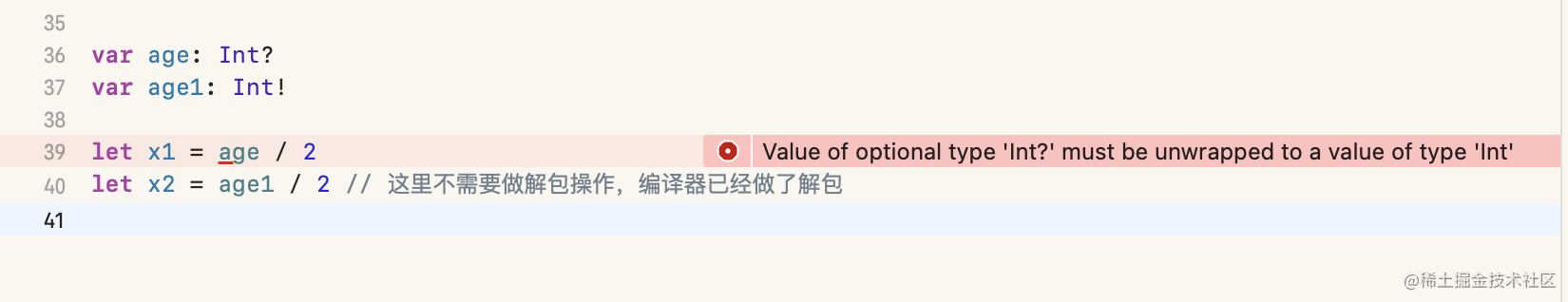在日常开发中比较常⻅这种隐士解析可选类型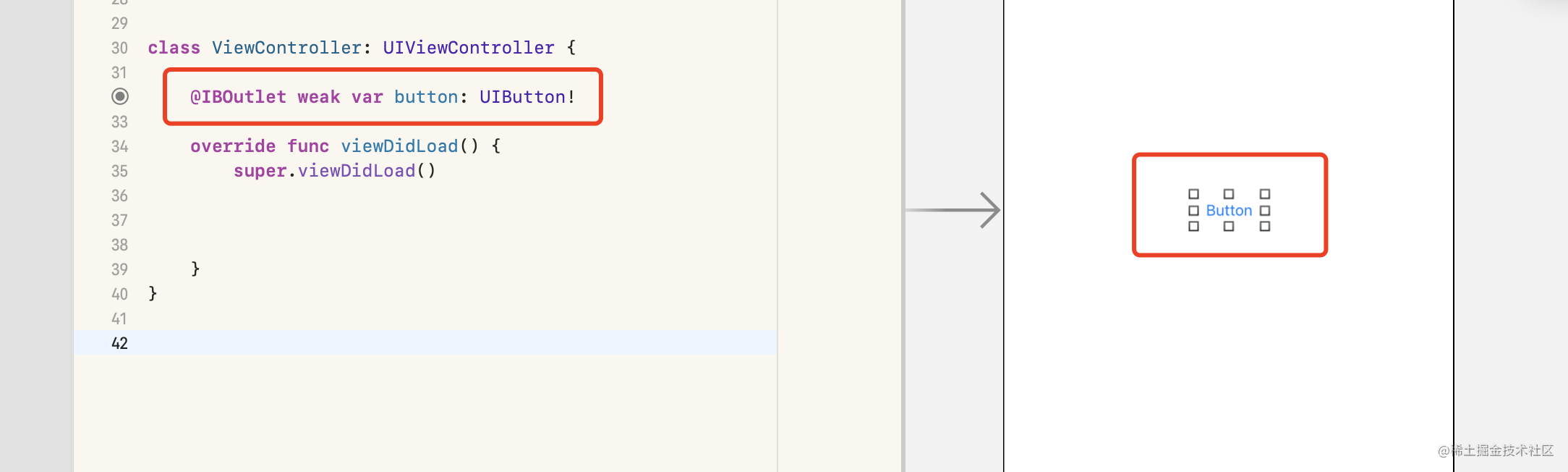`IBOutlet`类型是`Xcode`强制为可选类型的，因为它不是在初始化时赋值的，而是在加载视图的时候。你可以把它设置为普通可选类型，但是如果这个视图加载正确，它是不会为空的。

iOS